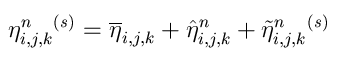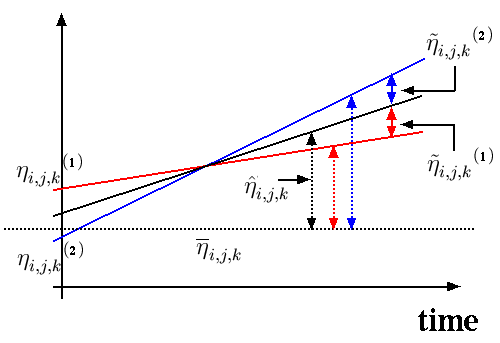Project information:

Resources:

## Variable separation in ensemble simulations

Consider a time series of one prognostic variable at one point i,j(,k) in x,y(,z)-space. There will be one such time series from each of the realizations in the ensemble. We separate the variable into three parts:Here, the overbar-trem is the overall mean value for all realizations, the hat-term is the deviation of the time series of ensemble means from the overall mean, and the tilde-term is the deviation of the time series of a particular realization from the time series of ensemble means.

For simplicity, assume that the ensemble consists of two realizations only. In the figure below, the time series for the chosen variable at a specific point in space is displayed by the red line (realization no. 1) and a blue line (realization no. 2). The full black line is the time series of the ensemble mean, and the dashed line is the overall mean value from the combined set time series (i.e., the time integrated mean).We interpret the deviation that corresponds to the hat-term (black dashed line with arrows) as a representation of the deterministic variability. Then, the variability corresponding to the tilde-term (red and blue solid lines with arrows) represents the non-deterministic variability. A mathematical expression relating these quantities to the variance of the time series is presented here.